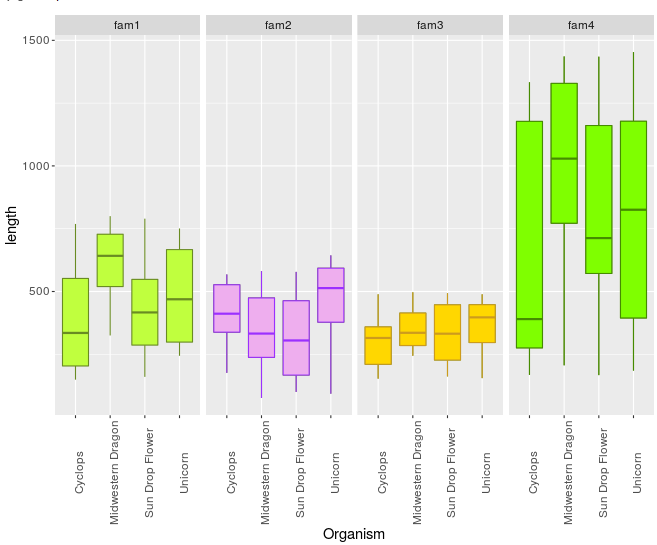## Using R to create boxplots

For datasets that have many observations per sample and you want to visualize their distribution, boxplots are good choice. Here, the example dataset has average values of multiple observations for each sample. It is organized in this way:

Table 1: Gene length for 4 different gene families and for 3 different organism.

Organism gene family 1 gene family 2 gene family 3 gene family 4
Unicorn 263 407 460 519
Unicorn 269 644 409 904
Unicorn 705 592 489 831
Unicorn 501 368 245 1269
Unicorn 244 520 403 227
Unicorn 387 275 280 185
Unicorn 437 93 391 353
Unicorn 552 612 476 1289
Unicorn 745 593 347 1453
Unicorn 751 507 155 820
Cyclops 583 463 153 1303
Cyclops 769 329 211 274
Cyclops 459 452 283 1289
Cyclops 149 568 210 280
Cyclops 181 314 412 1333
Cyclops 693 176 359 361
Cyclops 208 561 161 842
Cyclops 202 365 489 259
Cyclops 336 371 347 168
Cyclops 334 548 358 419
Midwestern Dragon 404 76 434 1188
Midwestern Dragon 325 466 259 1436
Midwestern Dragon 713 218 277 837
Midwestern Dragon 485 297 346 1258
Midwestern Dragon 652 527 325 750
Midwestern Dragon 632 342 309 1352
Midwestern Dragon 800 581 497 870
Midwestern Dragon 623 323 354 206
Midwestern Dragon 733 123 244 646
Midwestern Dragon 751 477 474 1390
Sun Drop Flower 743 100 473 625
Sun Drop Flower 160 230 164 1207
Sun Drop Flower 260 569 493 650
Sun Drop Flower 443 156 340 775
Sun Drop Flower 366 380 312 167
Sun Drop Flower 465 201 162 1435
Sun Drop Flower 576 488 440 1130
Sun Drop Flower 390 388 199 1171
Sun Drop Flower 164 115 324 554
Sun Drop Flower 790 578 449 530

If this table is in excel, we would want to export it as tab-delimited file in order to further manipulate using UNIX commands and then import it in R. For exporting: `File` > `Save As` > choose format `Text (Tab delimited)(*.txt)` and `Save`. Manipulating with UNIX commands: open the Lixnux sub-system (Windows) or Termina (Mac/Linux) and navigate to the directory the file is saved. If you are in Windows, you will need to run `dos2unix` to convert the line endings correctly. To format the above table to just 3 columns i.e,:

``````Organism  family  length
``````

following commands were used:

``````awk 'BEGIN{OFS=FS="\t"}{print \$1,"fam1",\$2}' test.txt | grep -v "Organism" > formatted.txt
awk 'BEGIN{OFS=FS="\t"}{print \$1,"fam2",\$3}' test.txt | grep -v "Organism">> formatted.txt
awk 'BEGIN{OFS=FS="\t"}{print \$1,"fam3",\$4}' test.txt | grep -v "Organism" >> formatted.txt
awk 'BEGIN{OFS=FS="\t"}{print \$1,"fam4",\$5}' test.txt | grep -v "Organism" >> formatted.txt
``````

Now we are ready to manipulate in R!

First, open the R prompt by typing `R` in the terminal, navigate the working directory, where the `formatted.txt` file is saved.

``````R
#then
setwd("path/to/your/directory")
``````

Import data and examine contents, format it if necessary:

``````file <- "formatted.txt"
#examine
names(input)
names(input) <- c("organism","family","length")
``````

If your data looks like this below, you’re all set:

``````> head(input)
Organism family length
1  Unicorn   fam1    263
2  Unicorn   fam1    269
3  Unicorn   fam1    705
4  Unicorn   fam1    501
5  Unicorn   fam1    244
6  Unicorn   fam1    387
>
``````

First, lets load all the required packages. We will need the `ggplot2` for plotting, `grid` package for making grids of plots/faceting.

``````library(ggplot2)
library(grid)
``````

For getting a simple boxplot, you can use the following command:

``````ggplot(input, aes(x=Organism,y=length)) + geom_boxplot()
``````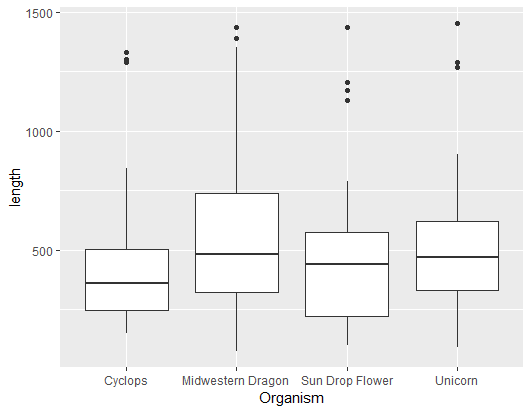if you want to color the plots,

``````ggplot(input, aes(x=Organism,y=length, fill=Organism)) + geom_boxplot()
``````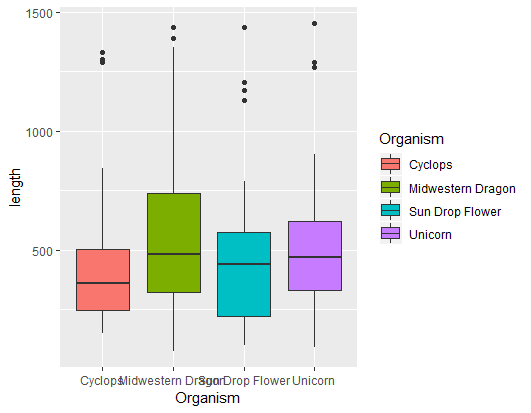but wait, where are the gene families?

``````ggplot(input, aes(x=Organism,y=length, fill=family)) + geom_boxplot()
``````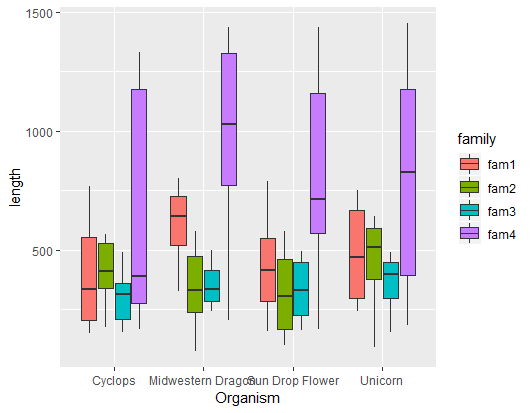That names are all overlapping though?

``````ggplot(input, aes(x=Organism,y=length, fill=family)) + geom_boxplot() + theme(axis.text.x = element_text(angle = 90))
``````Well, it seems like it is diffucult to compare as all gene families are together, can we spread them out in to separate plots?

Yes, but first we need to make different subsets that we want to plot

``````fam1 <- input[which(input\$family == "fam1"),]
fam2 <- input[which(input\$family == "fam2"),]
fam3 <- input[which(input\$family == "fam3"),]
fam4 <- input[which(input\$family == "fam4"),]
``````

Let’s also make colors to fill in for each family

``````fill1 <- "olivedrab1"
lines1 <- "olivedrab4"
fill2 <- "plum2"
lines2 <- "purple1"
fill3 <- "gold"
lines3 <- "goldenrod3"
fill4 <- "chartreuse"
lines4 <- "chartreuse4"
``````

plots for each family can be generated using dataframes for respective families:

``````ggplot(fam1, aes(x=Organism,y=length)) + geom_boxplot(colour = lines1, fill = fill1)
ggplot(fam2, aes(x=Organism,y=length)) + geom_boxplot(colour = lines2, fill = fill2)
ggplot(fam3, aes(x=Organism,y=length)) + geom_boxplot(colour = lines3, fill = fill3)
ggplot(fam4, aes(x=Organism,y=length)) + geom_boxplot(colour = lines4, fill = fill4)
``````

For a combined plots, arranged based on families:

Vertically

``````ggplot(input, aes(x=Organism,y=length)) + geom_boxplot() +
facet_grid( family ~ .)
#colored
ggplot(input, aes(x=Organism,y=length, fill=Organism)) +
geom_boxplot() +  facet_grid( family ~ .)
# accommodate long names
ggplot(input, aes(x=Organism,y=length, fill=Organism)) +
geom_boxplot() +  facet_grid( family ~ .)   +
theme(axis.text.x = element_text(angle = 90))

``````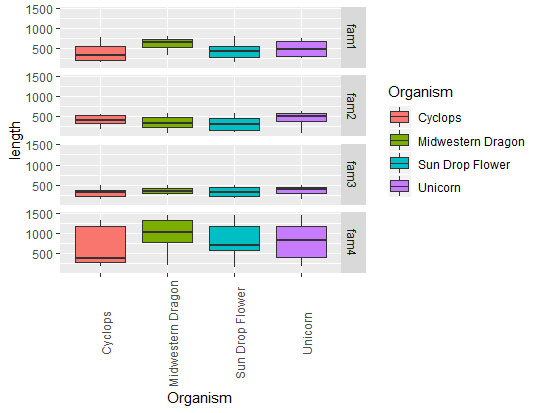Horizontally

``````ggplot(input, aes(x=Organism,y=length)) + geom_boxplot() +  facet_grid( . ~ family)
#since names are long ..
ggplot(input, aes(x=Organism,y=length)) +
geom_boxplot() +
facet_grid( .~ family) +
theme(axis.text.x = element_text(angle = 90))
# colored
ggplot(input, aes(x=Organism,y=length)) +
geom_boxplot(colour = lines1, fill = fill1) +
facet_grid( .~ family) +
theme(axis.text.x = element_text(angle = 90))
``````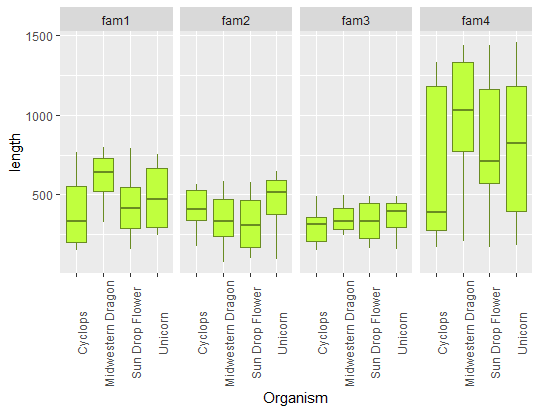To color these gene families:

``````p1 <- ggplot(input, aes(x=Organism,y=length)) +
+    geom_boxplot(colour = lines1, fill = fill1) +
+    facet_grid( .~ family) +
+    theme(axis.text.x = element_text(angle = 90))
p2 <- p1 + facet_wrap(~family)
p2 <- p1 + geom_boxplot(data = fam2, colour = lines2, fill = fill2)
p3 <- p2 + geom_boxplot(data = fam3, colour = lines3, fill = fill3)
p4 <- p3 + geom_boxplot(data = fam4, colour = lines4, fill = fill4)
``````